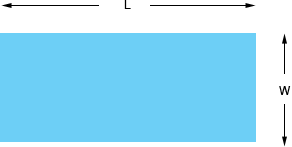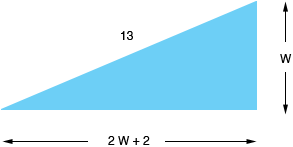Quandaries and Queries Name: Marcos Who is asking: Student Question: The length of a picture window is 2 feet longer than twice its width. If the diagonal of the window is 13 feet, find the dimensions of the window. Marcos, Draw a diagram and label it. I called the width W and the length L, both in feet.The instructions say that L is 2 feet longer than twice W, that is L = 2 W + 2 You also know that the diagonal is 13 feet long. Thus you haveThis is a right triangle. Do you know a relationship among the sides of a right triangle? Penny